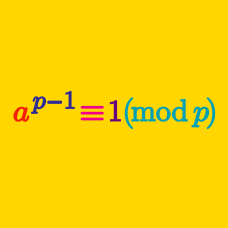Number Theory

# Chinese Remainder Theorem

If a positive integer $n$ satisfies $\left\{\begin{matrix} n \equiv 2 & \pmod{3}\\ n \equiv 3 & \pmod {5}, \end{matrix}\right.$ how many possible values of $n$ are in the domain $[10,29]?$

If a positive integer $n$ satisfies $\left\{\begin{matrix} n \equiv 2 & \pmod{5} \\ n \equiv 3 & \pmod {9} \\ n \equiv 1 & \pmod {11}, \end{matrix}\right.$ what is the $7 ^{\text{th}}$ smallest possible value of $n?$

What is the largest positive integer $n$ satisfying $\left\{\begin{matrix} n \equiv 1 & \pmod{3}\\ n \equiv 2 & \pmod {7}, \end{matrix}\right.$ in the domain $[0,600]?$

If we make a sequence with positive integers $n$ satisfying $\left\{\begin{matrix} n \equiv 2 & \pmod{3}\\ n \equiv 3 & \pmod {4} \end{matrix}\right.$ in increasing order, what is the $15 ^{\text{th}}$ term?

If $n \equiv 1 \pmod{3}$ and $n \equiv 3 \pmod { 7} ,$ what is $n?$

×